# Rotations of order 6, no reflections

The remaining patterns are numbered 101, which implies that one more further question needs to the answered.

The first group are examples of patterns with a hexagon. An example of two of these are: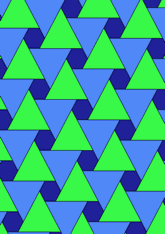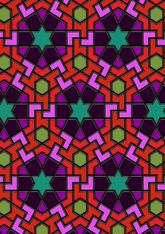The number in this group is 38, which is 37% of the total. Make your final selection, see page.
The second group are examples of patterns which have an equilateral triangle. An example of two of these are: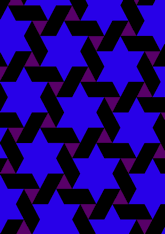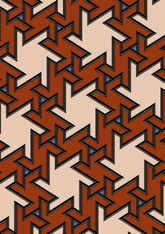The number in this group is 25, which is 24% of the total. Make your final selection, see page.
The last group are examples of patterns without either of the above. An example of two of these are: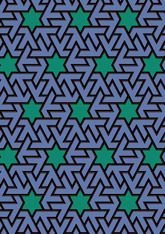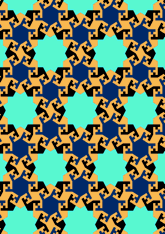The number in this group is 38, which is 37% of the total. Make your final selection, see page.

v53

Tiling Search was created by Brian Wichmann, and is maintained by MIT Libraries, through support from the Aga Khan Documentation Center.

Also supported by Performant Software Solutions LLC.